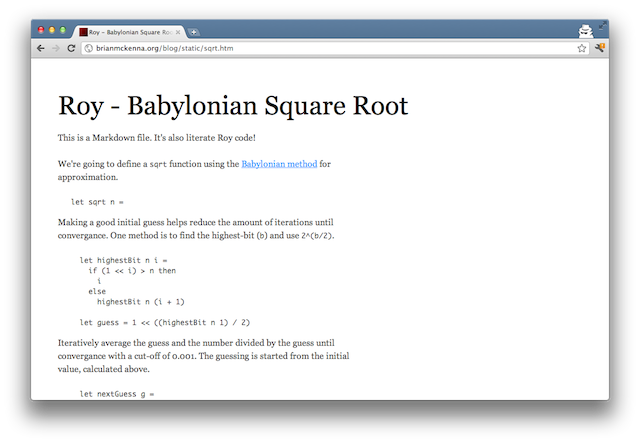## Roy - Literate Roy

I added some literate programming support to Roy. You can write Markdown files with an extension of `.lroy` and the `roy` executable will accept them.

I’ve also written a shell script that will run it through Pandoc to give you nice-looking HTML output, using Kevin Burke’s Markdown.css:The above was generated using the following Markdown:

``````% Roy - Babylonian Square Root

This is a Markdown file. It's also literate Roy code!

We're going to define a `sqrt` function using the
[Babylonian method][] for approximation.

let sqrt n =

Making a good initial guess helps reduce the amount of
iterations until convergence. One method is to find the
highest-bit (`b`) and use `2^(b/2)`.

let highestBit n i =
if (1 << i) > n then
i
else
highestBit n (i + 1)

let guess = 1 << ((highestBit n 1) / 2)

Iteratively average the guess and the number divided by the guess
until convergence with a cut-off of 0.001. The guessing is started
from the initial value, calculated above.

let nextGuess g =
if (Math.abs (g - (n / g))) < 0.001 then
g
else
nextGuess ((g + (n / g)) / 2)

nextGuess guess

Now we can calculate the square root of 314 and print it.

console.log (sqrt 314)

We should get an answer of around 17.720.

[Babylonian method]: http://en.wikipedia.org/wiki/Methods_of_computing_square_roots#Babylonian_method``````

Happy New Year!